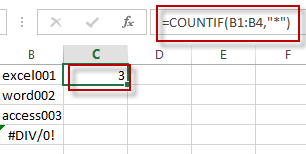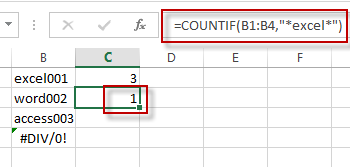# Count Cells That Contains Text or Specific Text

This post will guide you how to count the number of cells that contain text or the specific text in Excel. How do I count the number of cells if they contain text using excel formula.

## Count Cells That contains text

If you want to count the number of cells that contain text, you can use the COUNTIF function and a wildcard to create a new Excel Formula. Just like below this:

`=COUNTIF(B1:B4,"*")`The COUNTIF function will count the number of cells that match the given criteria. And the wildcard character will match any number of text characters.

If you want only to count the number of cells that contain specific text in a range, you still need to use the COUNTIF function with the specific characters and wildcard character. For example, to count the number of cells that contain “excel” text string, you can write down the following formula:

`=COUNTIF(B1:B4,"*excel*")`### Related Functions

• Excel COUNTIF function
The Excel COUNTIF function will count the number of cells in a range that meet a given criteria. This function can be used to count the different kinds of cells with number, date, text values, blank, non-blanks, or containing specific characters.etc.= COUNTIF (range, criteria)…